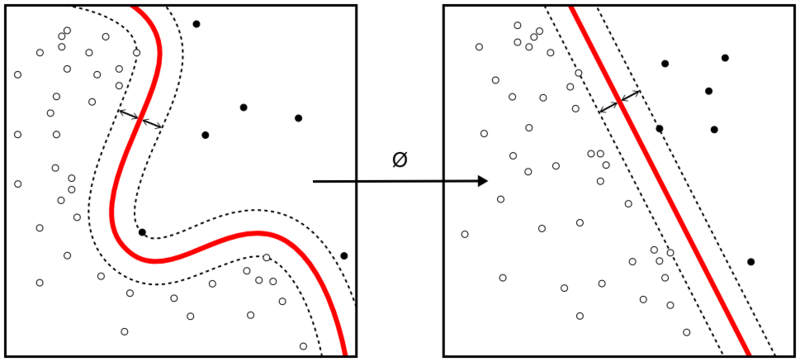## How to program the Support Vector Machines algorithm

Support Vector Machine is one of the most commonly used supervised machine learning algorithms for data classification. A binary classifier, the support vector machine algorithm works in vector space to sort data points by finding the best hyperplane separating them into two groups. Thanks to its reliance upon vectors, it finds frontiers between groups of data points even in nonlinear patterns and features spaces of high dimensions.Continue reading “How to program the Support Vector Machines algorithm”

## How to program the K Nearest Neighbors algorithm

K Nearest Neighbors is a popular classification algorithm for supervised machine learning. It permits to divide data points into groups, defining a model that will then be able to classify an unknown data point in one group or another. The K parameter, defined during programming, allows the algorithm to classify unknown data points by examining the K closest known data points.Continue reading “How to program the K Nearest Neighbors algorithm”

## Conceptual and mathematical summary for machine learning

Machine learning makes use of multiple mathematical formulas and relations to implement the different tasks it can handle. Gathered in the following “cheat sheets” by Afshine and Shervine Amidi, the concepts for supervised and unsupervised learning, deep learning together with machine learning tips and tricks, probabilities, statistics algebra and calculus reminders, are all presented in details with the underlying math.Based on the Stanford course on Machine Learning (CS 229), the cheat sheets summarize the important concepts of each branch with simple explanations and diagrams, such as the following table cover underfitting and overfitting.

 Underfitting Just right Overfitting Symptoms • High training error • Training error close to test error • High bias • Training error slightly lower than test error • Very low training error • Training error much lower than test error • High variance Regression illustrationClassification illustrationDeep learning illustrationPossible remedies • Complexify model • Add more features • Train longer • Perform regularization • Get more data

The main machine learning cheat sheets can be found here:

Other mathematics and coding cheat sheets can be found here:

The complete cheat sheets can also be found on Github.